Complement of set

Chapter 1 Class 11 Sets
Concept wise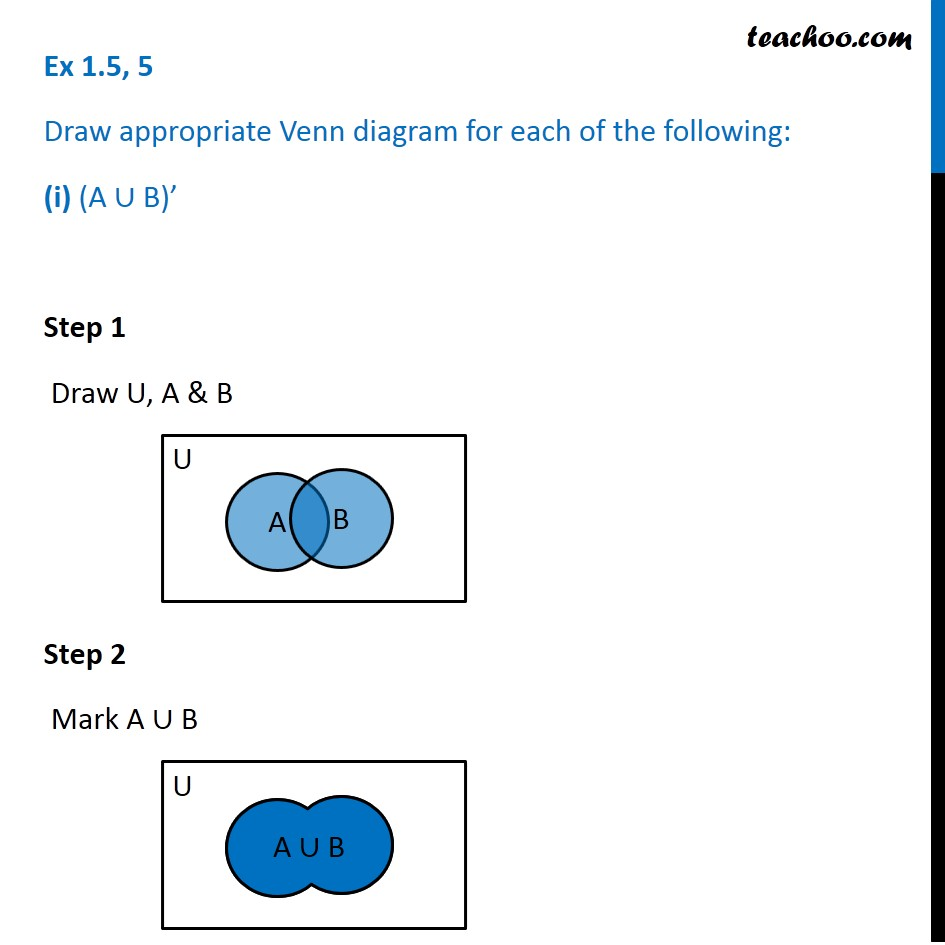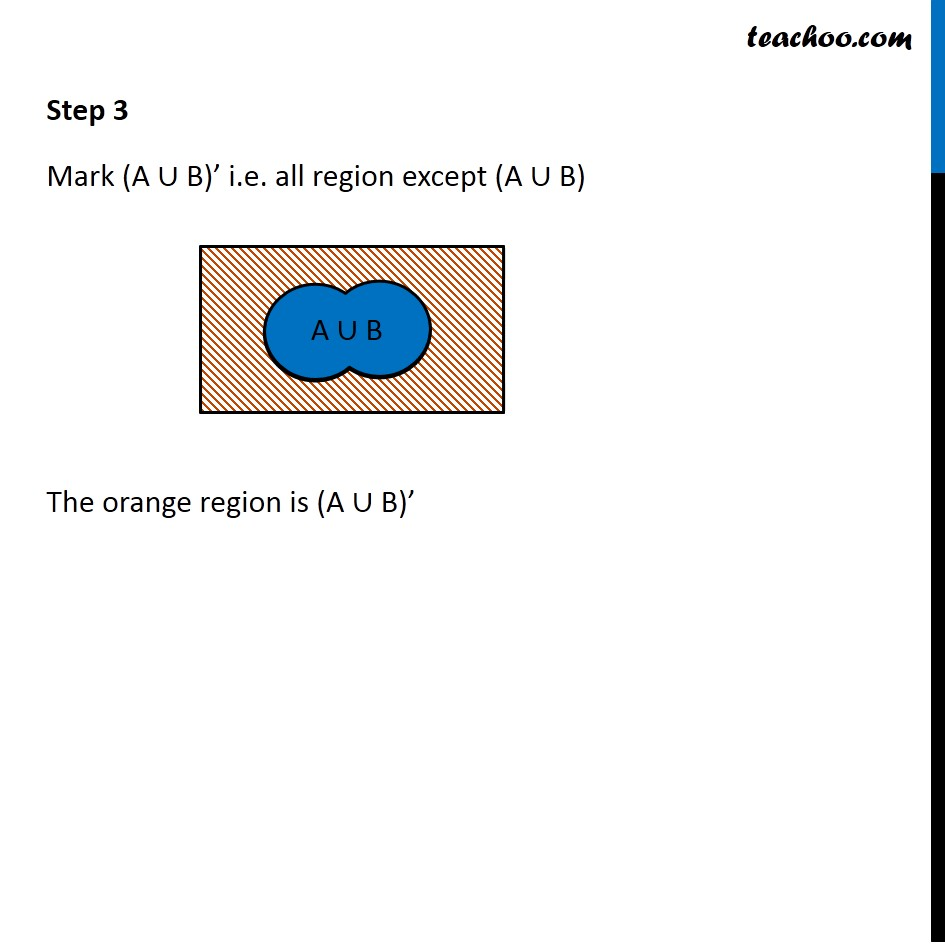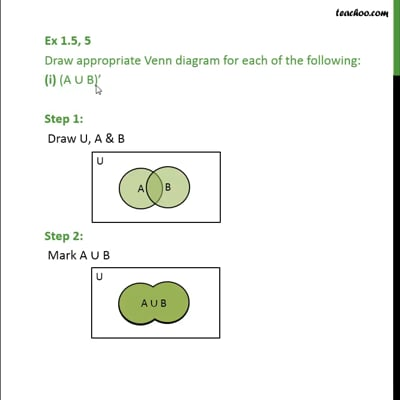This video is only available for Teachoo black users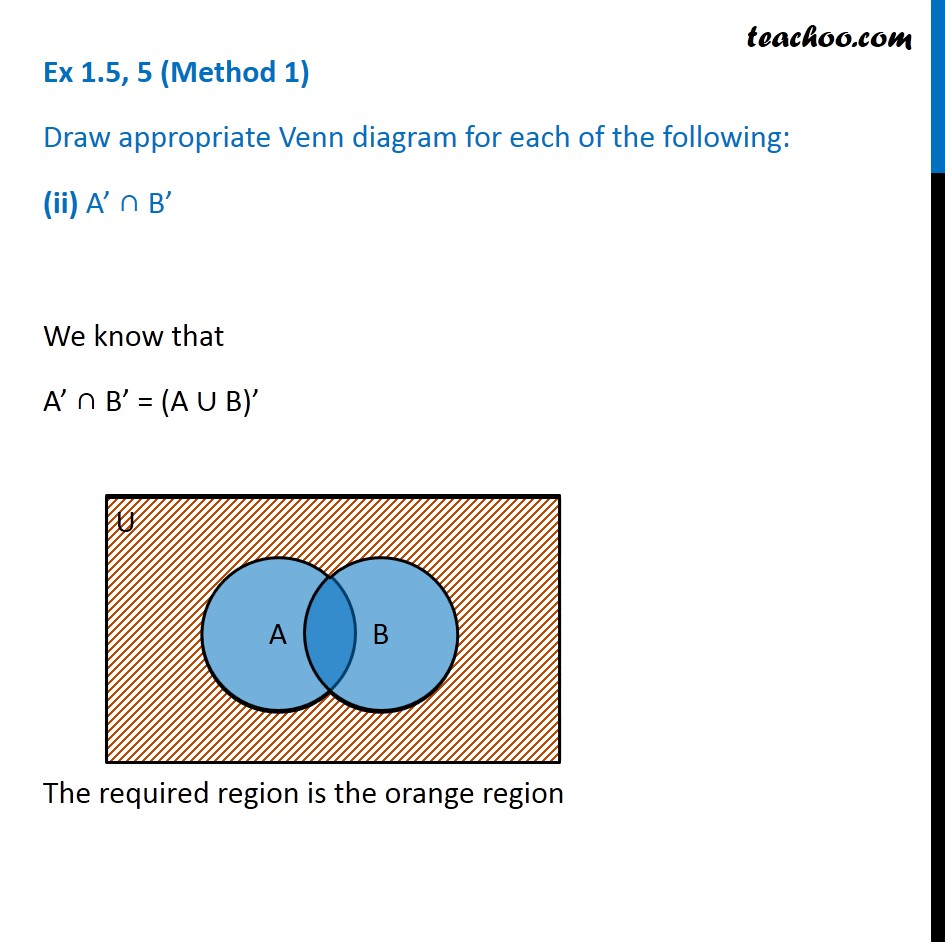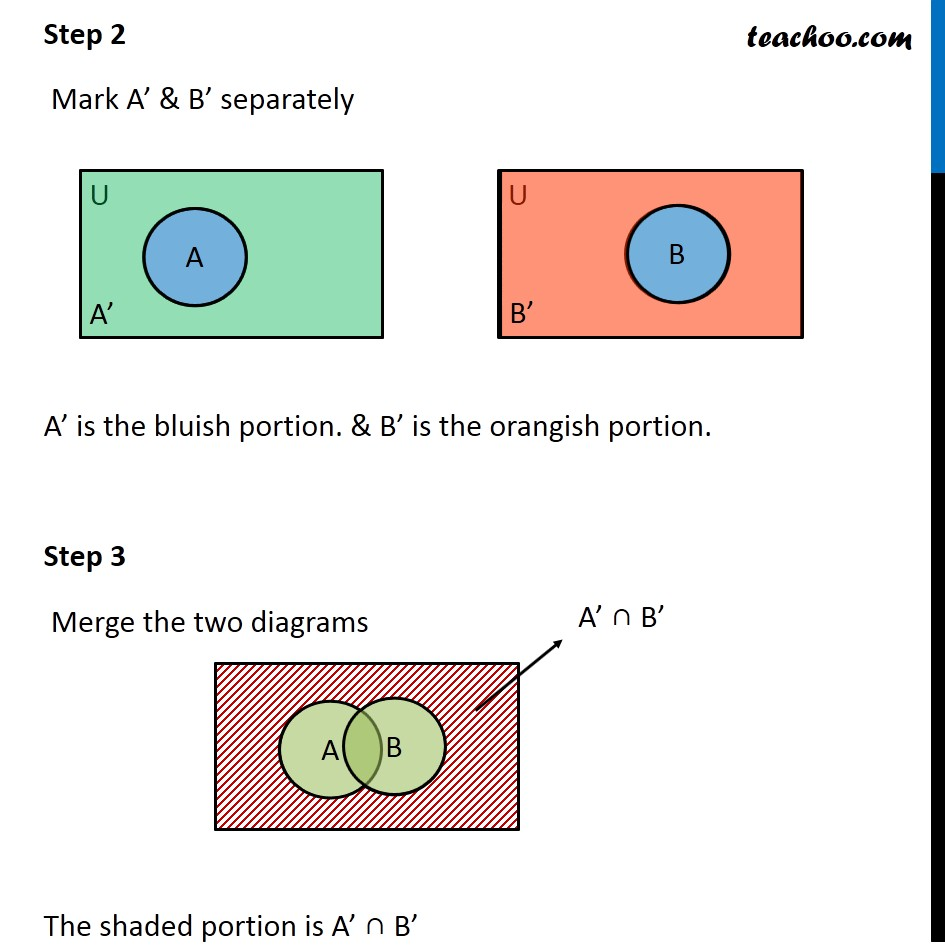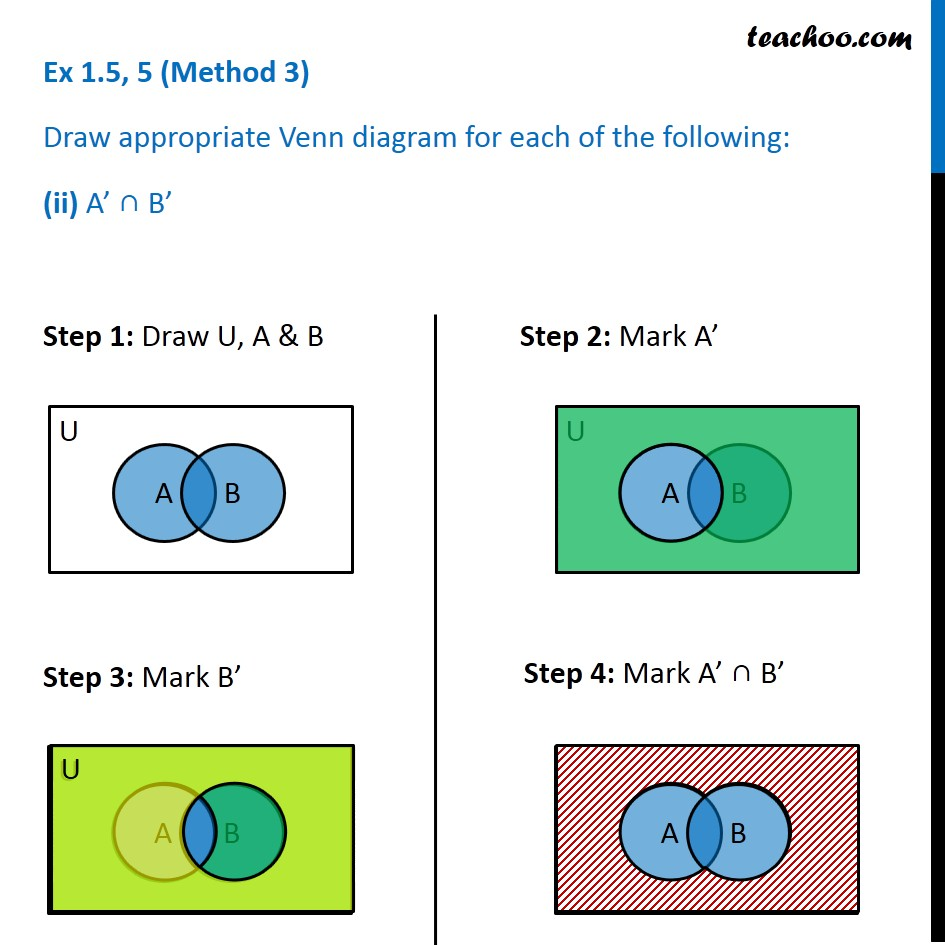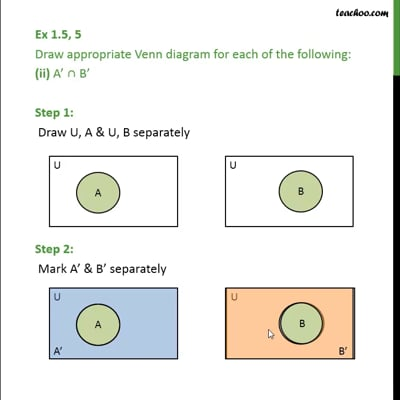This video is only available for Teachoo black users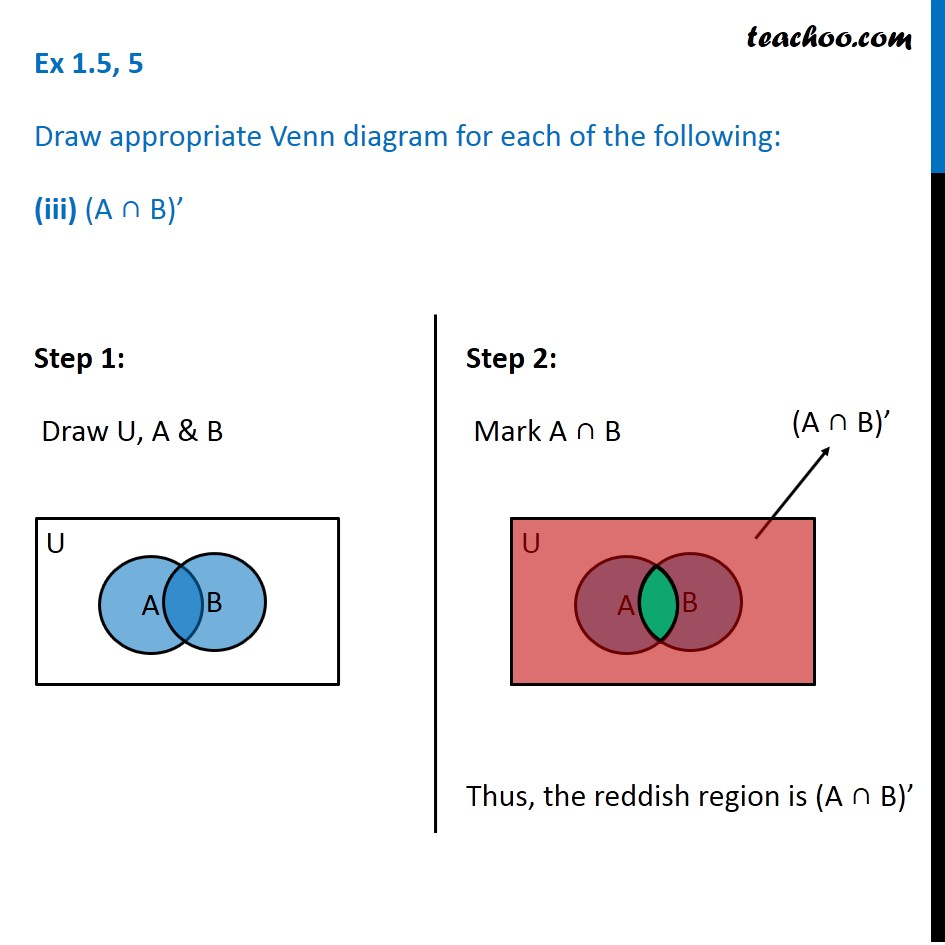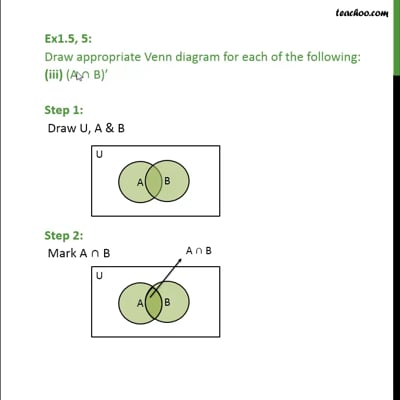This video is only available for Teachoo black users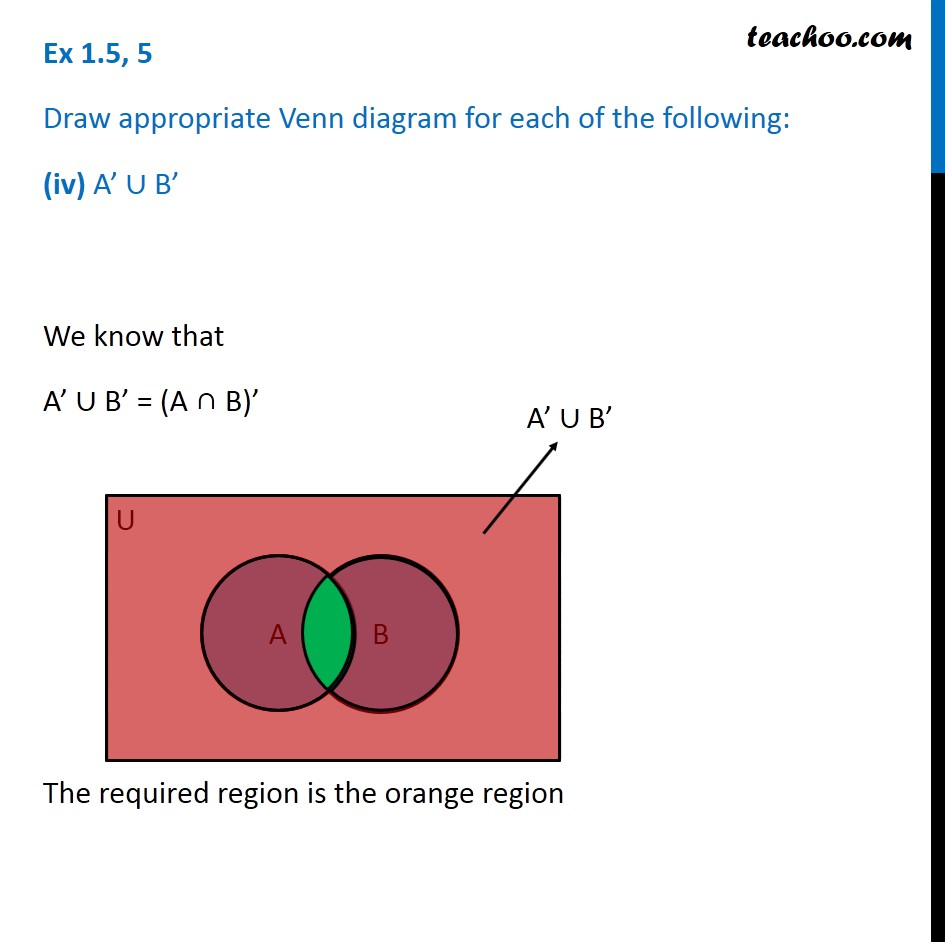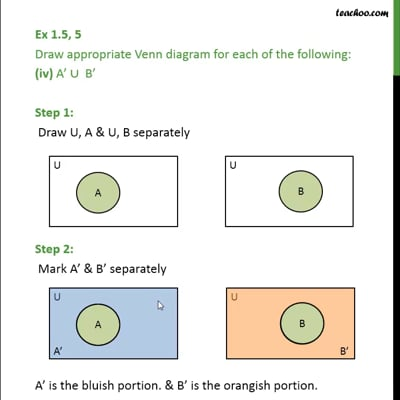This video is only available for Teachoo black users

Introducing your new favourite teacher - Teachoo Black, at only ₹83 per month

### Transcript

Ex 1.5, 5 Draw appropriate Venn diagram for each of the following: (i) (A ∪ B)’ Step 1 Draw U, A & B Step 2 Mark A ∪ B Step 3 Mark (A ∪ B)’ i.e. all region except (A ∪ B) The orange region is (A ∪ B)’ Ex 1.5, 5 (Method 1) Draw appropriate Venn diagram for each of the following: (ii) A’ ∩ B’ We know that A’ ∩ B’ = (A ∪ B)’ The required region is the orange region Ex 1.5, 5 (Method 2) Draw appropriate Venn diagram for each of the following: (ii) A’ ∩ B’ Step 1 Draw U, A & U, B separately Step 2 Mark A’ & B’ separately A’ is the bluish portion. & B’ is the orangish portion. Step 3 Merge the two diagrams The shaded portion is A’ ∩ B’ Ex 1.5, 5 (Method 3) Draw appropriate Venn diagram for each of the following: (ii) A’ ∩ B’ Step 1: Draw U, A & B Step 2: Mark A’ Ex 1.5, 5 Draw appropriate Venn diagram for each of the following: (iii) (A ∩ B)’ Step 1: Draw U, A & B Step 1: Draw U, A & B Ex 1.5, 5 Draw appropriate Venn diagram for each of the following: (iv) A’ ∪ B’ We know that A’ ∪ B’ = (A ∩ B)’ The required region is the orange region